Next: Calculation of Clebsch-Gordon Coefficients Up: Addition of Angular Momentum Previous: Commutation Rules

# Clebsch-Gordon Coefficients

As we have seen, the operator group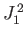,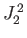,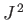, and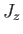is incompatible with the group,,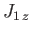, and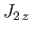. This means that if the system is in a simultaneous eigenstate of the former group then, in general, it is not in a simultaneous eigenstate of the latter. In other words, if the quantum numbers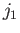,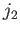,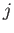, and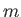are known with certainty then a measurement of the quantum numbers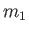and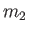will give a range of possible values. We can use the completeness relation (6.20) to write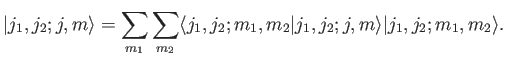(6.22)

Thus, we can write the eigenkets of the first group of operators as a weighted sum of the eigenkets of the second set. The weights,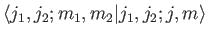, are called the Clebsch-Gordon coefficients. If the system is in a state where a measurement of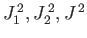, andis bound to give the results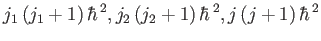, and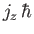, respectively, then a measurement ofandwill give the results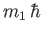and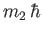, respectively, with probability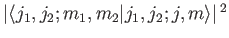.

The Clebsch-Gordon coefficients possess a number of very important properties. First, the coefficients are zero unless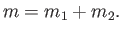(6.23)

To prove this, we note that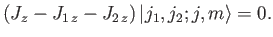(6.24)

Forming the inner product with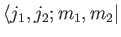, we obtain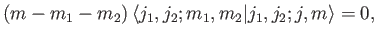(6.25)

which proves the assertion. Thus, the-components of different angular momenta add algebraically. So, an electron in anstate, with orbital angular momentum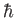, and spin angular momentum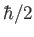, projected along the-axis, constitutes a state whose total angular momentum projected along the-axis is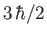. What is uncertain is the magnitude of the total angular momentum.

Second, the coefficients vanish unless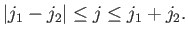(6.26)

We can assume, without loss of generality, that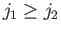. We know, from Equation (6.23), that for givenandthe largest possible value ofis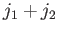(becauseis the largest possible value of, etc.). This implies that the largest possible value ofis(because, by definition, the largest value ofis equal to). Now, there are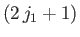allowable values of, and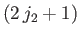allowable values of. Thus, there are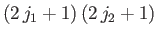independent eigenkets,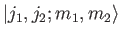, needed to span the ket space corresponding to fixedand. Because the eigenkets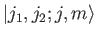span the same space, they must also form a set ofindependent kets. In other words, there can only bedistinct allowable values of the quantum numbersand. For each allowed value of, there are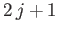allowed values of. We have already seen that the maximum allowed value ofis. It is easily seen that if the minimum allowed value ofis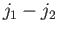then the total number of allowed values ofandis. In other words ,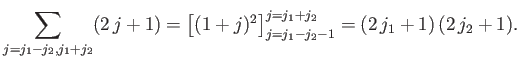(6.27)

This proves our assertion.

Third, the sum of the modulus squared of all of the Clebsch-Gordon coefficients is unity: that is,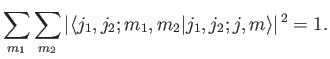(6.28)

This assertion is proved as follows: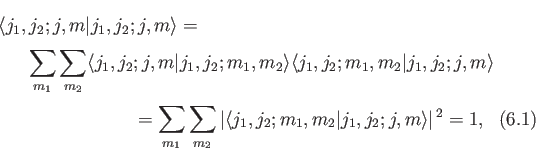where use has been made of the completeness relation (6.20).

Finally, the Clebsch-Gordon coefficients obey two recursion relations. To obtain these relations, we start from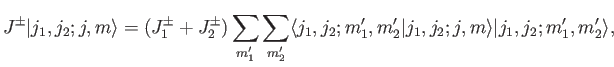(6.29)

where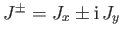, et cetera, Making use of the well-known properties of the ladder operators,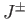,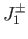, and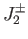, which are specified by analogy with Equations (4.55)-(4.56), we obtain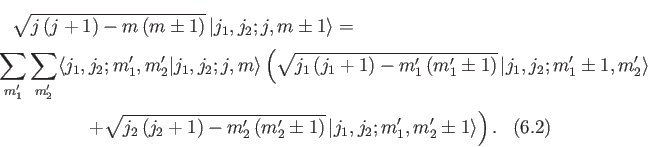Taking the inner product with, and making use of the orthonormality property of the basis eigenkets, we get the desired recursion relations: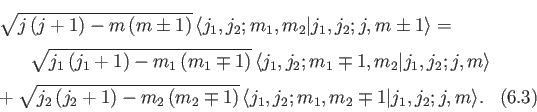It is clear, from the absence of complex coupling coefficients in the previous relations, that we can always choose the Clebsch-Gordon coefficients to be real numbers. This is convenient, because it ensures that the inverse Clebsch-Gordon coefficients,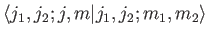, are identical to the Clebsch-Gordon coefficients. In other words,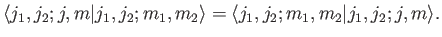(6.30)

The inverse Clebsch-Gordon coefficients are the weights in the expansion of thein terms of the: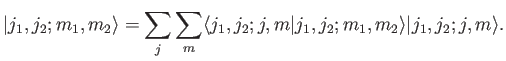(6.31)

It turns out that the recursion relations (6.32), together with the normalization condition (6.28), are sufficient to completely determine the Clebsch-Gordon coefficients to within an arbitrary sign (multiplied into all of the coefficients). This sign is fixed by convention. [To be more exact, each Clebsch-Gordon sub-table associated with a specific value of(see later) is undetermined to an arbitrary sign. It is conventional to give the Clebsch-Gordon coefficient with the largest value ofa positive sign.] The easiest way of demonstrating this assertion is by considering a specific example.Next: Calculation of Clebsch-Gordon Coefficients Up: Addition of Angular Momentum Previous: Commutation Rules
Richard Fitzpatrick 2016-01-22